[Next] [Previous] [Up] [Top]

NUMERICAL METHOD FOR SPECIATION AND FORWARD MODELING

## Application to Initial Surface Calculations

A limited set of equations is included in initial surface calculations, that is, when the composition of a surface assemblage is defined to be that which is in equilibrium with a specified solution composition. The Newton-Raphson equations for the initial surface calculation are derived from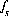,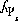or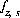,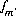,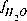, and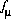, which are equations for mole-balance equations for each type of surface site in the surface assemblage, charge-potential or charge balance for each surface (both of these equations are excluded in the non-electrostatic model), mole balance for each element or element valence state, activity of water, and ionic strength. For initial surface calculations, the values of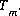include only the aqueous concentrations and the corresponding mole-balance equationsdo not contain terms for the contribution of the surfaces to the total element concentrations. The values calculated for all quantities related to the aqueous phase are the same as for the solution without the surface assemblage present.

For the explicit calculation of the diffuse layer, a charge-balance equation is used for each surface,; the values of the master unknowns for each surface of the surface assemblage,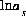and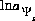, are adjusted to achieve mole balance and charge balance for each surface. If the diffuse-layer composition is not explicitly included in the calculation, then the charge-potential equation,, is used in place of the surface charge-balance equation. If the non-electrostatic model is used for the surface assemblage, then neither the surface charge balance nor the charge-potential equation is included in the set of equations to be solved.

All equations for initial surface calculations are included as equality constraints in the solver. No equations are optimized and no inequality constraints are included.

An initial surface calculation is performed only if the surface initially is defined to be in equilibrium with a specified solution. The distribution of species for this solution has already been calculated, either by an initial solution calculation or by a reaction or transport calculation. Thus, the initial estimates of all master unknowns related to the aqueous phase are set equal to the values from the previous distribution of species. The initial estimate of the activity of the master species for each surface is set equal to one tenth of the number of moles of surface sites for that surface. For explicit and implicit diffuse-layer calculations, the initial estimate of the potential unknown,, for each surface is zero, which implies that the surface potential is zero.

For data input to PHREEQC, definition of the initial surface calculation is made with the SURFACE keyword data block. (See Description of Data Input.)

User's Guide to PHREEQC - 07 MAY 96
[Next] [Previous] [Up] [Top]